# Solved Questions Structure of Atom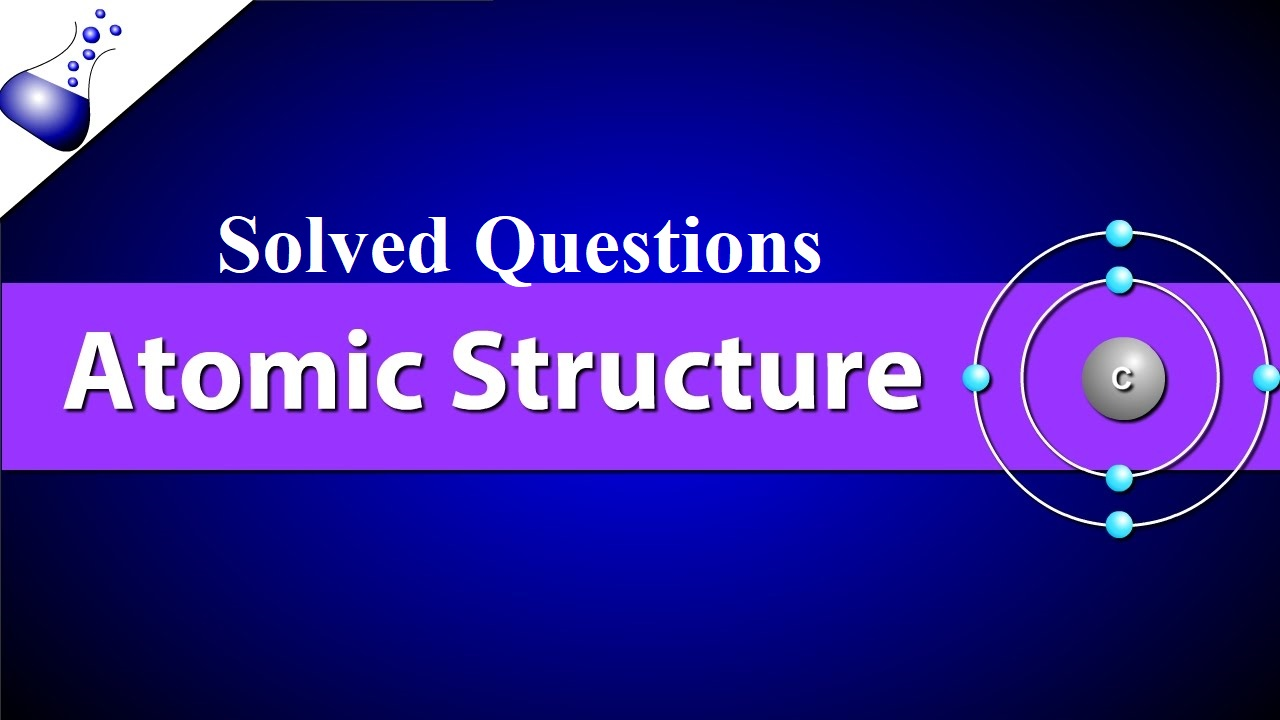Solved Questions Structure of Atom

Que 1. Neutrons can be found in all atomic nuclei except in one case. Which is this atomic nucleus and what does it consists of?
Ans 1. Hydrogen atom. It consists of only one proton.

Que 2. Calculate wave number of yellow radiations having a wavelength of 5800 A0.
Ans 2. Wave number = 1/ wavelength
Wavelength = 5800 A0= 5800 x 10-10 m
Wave number = 1/5800 x 10-10 m = 1.72 x 106 m-1

Que 3. What are the values of n and l for 2p orbital?
Ans 3. n=2 and l= 1

Que 4. Which of the following orbitals are not possible? 1p, 2s, 3f and 4d
Ans 4. 1p and 3f are not possible.

Que 5. Write the electronic configuration of the element having atomic number 24.
Ans 5. 1s2 2s2 2p6 3s2 3p6 3d5 4s1

Que 6. What is the relationship between frequency and wavelength of light?
Ans 6. velocity of light = frequency x wavelength. Frequency and wavelength are inversely proportional to each other.

Que 7. State Pauli Exclusion Principle.
Ans 7. No two electrons in an atom can have the same set of four quantum numbers or an orbital can have maximum two electrons and these must have opposite spin.

Que 8. When α- rays hit a thin foil of gold, very few α- particles are deflected back. What does it prove?
Ans 8. There is a very small heavy body present within the atom.

Que 9. What is the difference between a quantum and a photon?
Ans 9. The smallest packet of the energy of any radiation is called a quantum whereas that of light is called a photon.

Que 10. Using s, p, d and f notation, describe the orbital with the following quantum numbers-
(a) n = 1, l = 0     (b) n = 3, l = 1            (c) n = 4, l = 2             (d) n = 4, l = 3
Ans 10. (a) 1s (b) 3p (c) 4d (d) 4f

Que 11. How many electrons in an atom have the following quantum numbers?
(a) n = 4, m = -1/2         (b) n = 3 , l = 0
Ans 11. (a) 16 electrons  (b) 2 electrons.

Numerical Portion of Solved Questions Structure of Atom

Que 12. An element with mass number 81 contains 31.7 % more neutrons as compared to protons. Assign the atomic symbol.
Ans 12. Mass number = 81, i.e., p + n = 81
If protons = x, then neutrons = x + 31.7 × x = 1.317 x
100
x+1.317x = 81 or 2.317x = 81     x=35
Thus proton = 35, i.e., atomic no. = 35   Hence symbol is 8135Br

Que 13. (i) The energy associated with the first orbit in the hydrogen atom is -2.18 × 10-18J/atom. What is the energy associated with the fifth orbit?
(ii) Calculate the radius of Bohr’s fifth orbit for the hydrogen atom.
Ans 13. (i) En = -2.18 × 10-18/ n2           E5 = -2.18 × 10-18/ 52   = -8.72 × 10-20 J
(ii) For H atom, rn= 0.529 × n2         r5 = 0.529 × 52 = 13.225 A0= 1.3225 nm

Que 14. Calculate the total number of angular nodes and radial nodes present in 3p orbitals.
Ans 14. For 3p orbitals, n=3, l= 1
Number of angular nodes = l= 1
Number of radial nodes = n-l-1 = 3-1-1= 1

Que 15. Write the 3 points of difference between orbit and orbital.
Ans 15.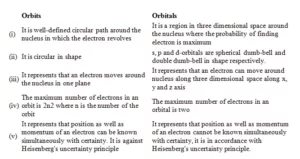Que 16. State Heisenberg’s uncertainty principle. Calculate the uncertainty in the position of an electron if the uncertainty in its velocity is 5.7 × 105 m/s.
Ans 16. It states that it is impossible to determine simultaneously, the exact position and exact momentum (or velocity) of an electron.  The product of their uncertainties is always equal to or greater than h/4π.   Δx  × (m × Δv) = h/4ᴨ
Δx = h/4ᴨ × m × Δv
= 6.6 × 10-34/4 × 3.14 × 9.1 × 10-31 × 5.7 × 105 = 1.0 × 10-10 m
Que 17.  (i) Calculate the number of electrons that will together weigh one gram.
(ii) Calculate the mass and charge of one mole of electrons
Ans 17. (i) Mass of one electron = 9.10939 × 10–31 kg
Number of electrons that weigh 9.10939 × 10–31 kg = 1
Number of electrons that will weigh 1 g = (1 × 10–3kg)
=                1×10-3
9.10939 × 10–31 kg
= 0.1098 × 10–3 + 31
= 0.1098 × 1028
= 1.098 × 1027
(ii) Mass of one electron = 9.10939 × 10–31 kg
Mass of one mole of electron = (6.022 × 1023) × (9.10939 ×10–31 kg)
= 5.48 × 10–7 kg
Charge on one electron = 1.6022 × 10–19coulomb
Charge on one mole of electron = (1.6022 × 10–19C) (6.022 × 1023)
= 9.65 × 104 C
Que 18. Find the energy of each of the photons which
(I) Correspond to the light of frequency 3× 1015Hz.
(ii) Have a wavelength of 0.50 Å.
Ans 18. (i) Energy (E) of a photon is given by the expression,
E =  h ν
Where,  h = Planck’s constant = 6.626 × 10–34Js
ν = frequency of light = 3 × 1015Hz
Substituting the values in the given expression of E
E = (6.626 × 10–34) (3 × 1015)
E = 1.988 × 10–18J
(ii) Energy (E) of a photon having wavelength (λ)is given by the expression,
E=hc/ λ
h = Planck’s constant = 6.626 × 10–34Js
c = velocity of light in vacuum = 3 × 108m/s Substituting the values in the given expression of E:
E= 6.626 × 10–34 × 3 × 108
0.50 × 10-10
E = 3.976 × 10-15 J
E = 3.98 × 10-15 J
Que 19. What is the wavelength of light emitted when the electron in a hydrogen atom undergoes a transition from an energy level with n = 4 to an energy level with n = 2?
Ans 19.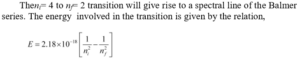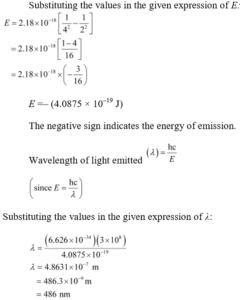Que 20. An atom of an element contains 29 electrons and 35 neutrons. Deduce
(i) The number of protons and
(ii) The electronic configuration of the element
(iii) Identify the element.
Ans 20.
(i) For an atom to be neutral, the number of protons is equal to the number of electrons.  ∴ Number of protons in the atom of the given element = 29
(ii) The electronic configuration of the atom is 1s22s2 2p6 3s2 3p64s2 3d10
(iii) Copper

Que 21. Give the number of electrons in the species H2+, H2, and O2+
Ans 21. Number of electrons present in hydrogen molecule (H2) = 1 + 1 = 2
∴ Number of electrons in H2+ = 2 – 1 = 1
Number of electrons in H2 = 1 + 1 = 2
Number of electrons present in oxygen molecule (O2) = 8 + 8 = 16
∴ Number of electrons in O2+ = 16 – 1 = 15

Que 22. State photoelectric effect. The work function for the cesium atom is 1.9 eV. Calculate
(a) the threshold wavelength
(b) the threshold frequency of the radiation. If the cesium element is irradiated with a wavelength of 500 nm, Calculate the kinetic energy and the velocity of the ejected photoelectron.
Ans 22. Photoelectric effect: The phenomenon of ejection of electrons from the surface of the metal when the light of suitable frequency strikes it is called the photoelectric effect. The ejected electrons are called photoelectrons.  It is given that the work function (W0) for cesium atom is 1.9 eV.
(a)From the expression,  W0 = hc/ λ0, we get:
λ0 = hc/ W0
Where, λ0 = threshold wavelength  h = Planck’s constant c = velocity of radiation Substituting the values in the given expression of (λ0):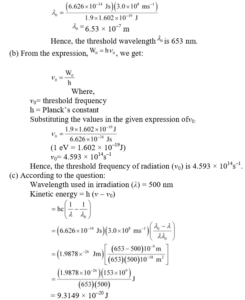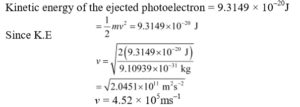Solved Questions Structure of Atom